This is “Solving Radical Equations”, section 8.6 from the book Beginning Algebra (v. 1.0). For details on it (including licensing), click here.

Has this book helped you? Consider passing it on:
Creative Commons supports free culture from music to education. Their licenses helped make this book available to you.
DonorsChoose.org helps people like you help teachers fund their classroom projects, from art supplies to books to calculators.

### Learning Objectives

1. Solve equations involving square roots.
2. Solve equations involving cube roots.

A radical equationAny equation that contains one or more radicals with a variable in the radicand. is any equation that contains one or more radicals with a variable in the radicand. Following are some examples of radical equations, all of which will be solved in this section:We begin with the squaring property of equalityGiven real numbers a and b, where $a=b$, then $a2=b2$.; given real numbers a and b, we have the following:In other words, equality is retained if we square both sides of an equation.The converse, on the other hand, is not necessarily true:This is important because we will use this property to solve radical equations. Consider a very simple radical equation that can be solved by inspection:Here we can see that $x=9$ is a solution. To solve this equation algebraically, make use of the squaring property of equality and the fact that $(a)2=a2=a$ when a is positive. Eliminate the square root by squaring both sides of the equation as follows:As a check, we can see that $9=3$ as expected. Because the converse of the squaring property of equality is not necessarily true, solutions to the squared equation may not be solutions to the original. Hence squaring both sides of an equation introduces the possibility of extraneous solutionsA solution that does not solve the original equation., or solutions that do not solve the original equation. For this reason, we must check the answers that result from squaring both sides of an equation.

Example 1: Solve: $x−1=5$.

Solution: We can eliminate the square root by applying the squaring property of equality.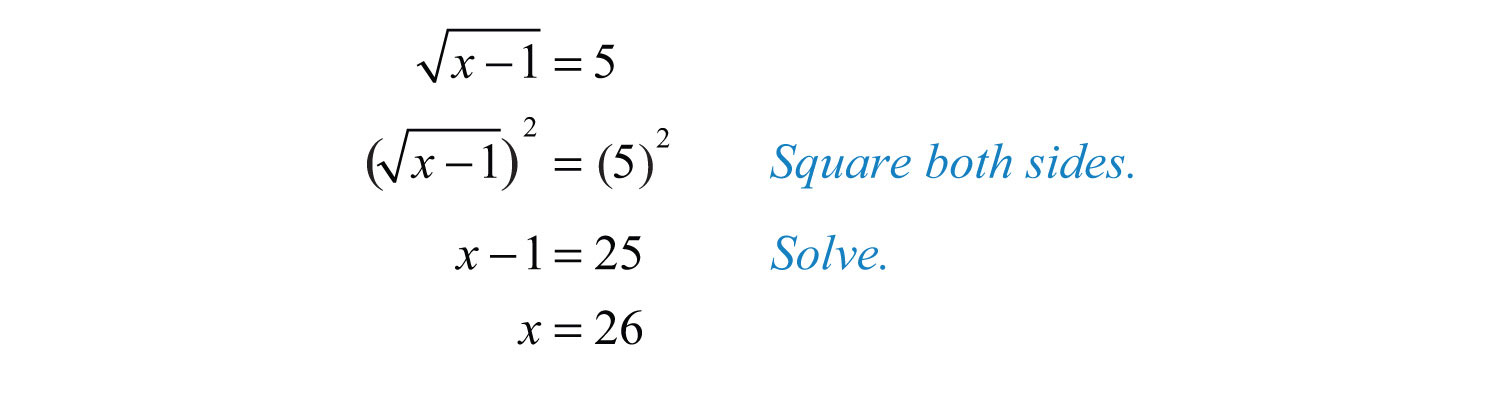Next, we must check.Example 2: Solve: $5−4x=x$.

Solution: Begin by squaring both sides of the equation.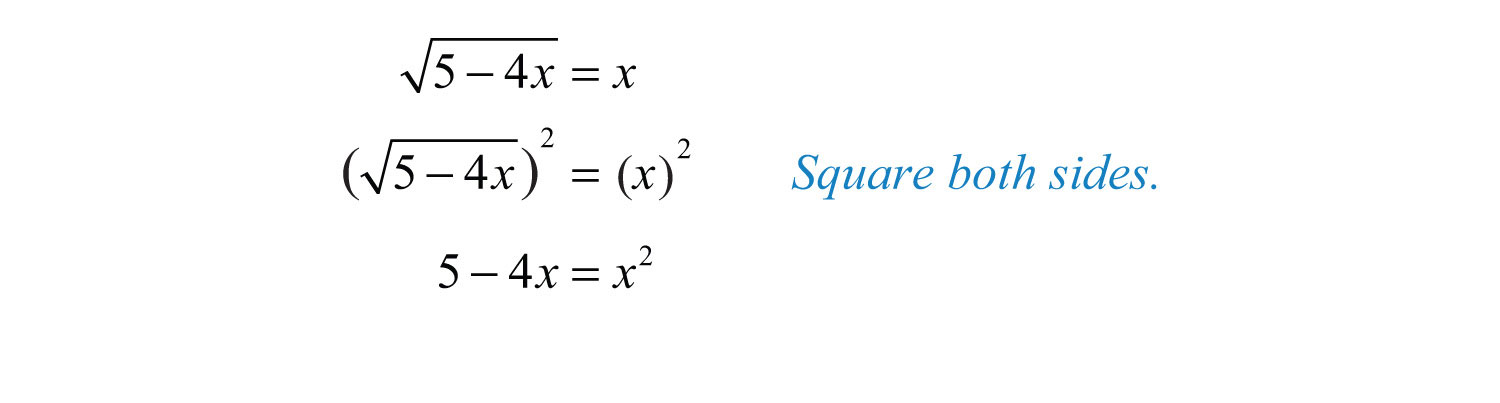You are left with a quadratic equation that can be solved by factoring.Since you squared both sides, you must check your solutions.After checking, you can see that $x=−5$ was extraneous; it did not solve the original radical equation. Disregard that answer. This leaves $x=1$ as the only solution.

Answer: The solution is $x=1$.

In the previous two examples, notice that the radical is isolated on one side of the equation. Typically, this is not the case. The steps for solving radical equations involving square roots are outlined in the following example.

Example 3: Solve: $2x−5+4=x$.

Solution:

Step 1: Isolate the square root. Begin by subtracting 4 from both sides of the equation.Step 2: Square both sides. Squaring both sides eliminates the square root.Step 3: Solve the resulting equation. Here you are left with a quadratic equation that can be solved by factoring.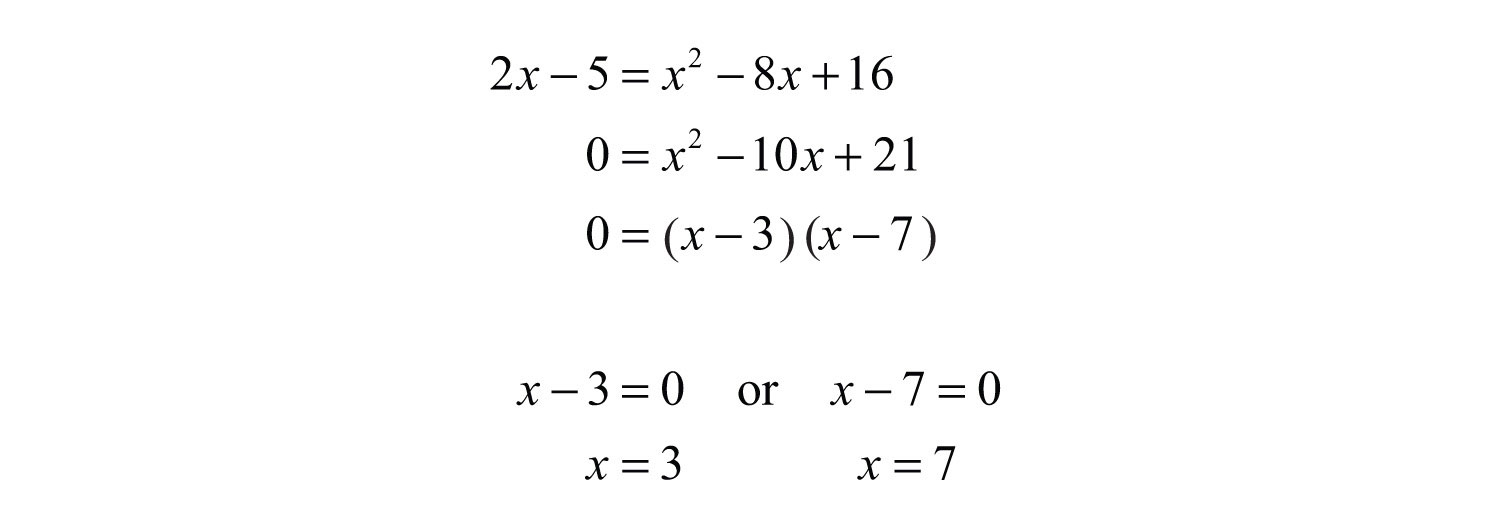Step 4: Check the solutions in the original equation. Squaring both sides introduces the possibility of extraneous solutions; hence the check is required.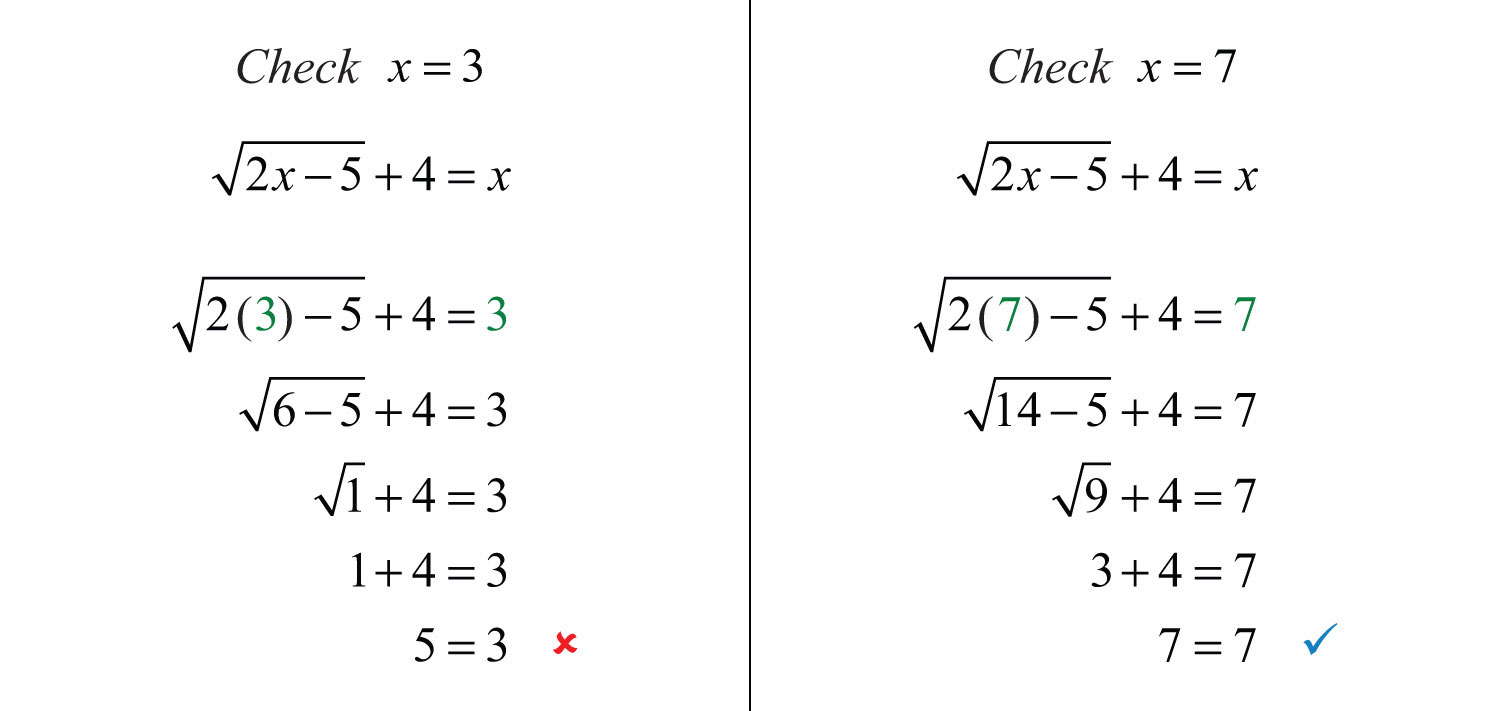After checking, we can see that $x=3$ is an extraneous root; it does not solve the original radical equation. This leaves $x=7$ as the only solution.

Answer: The solution is $x=7$.

Example 4: Solve: $3x+1−2x=0$.

Solution: Begin by isolating the term with the radical.Despite the fact that the term on the left side has a coefficient, it is still considered isolated. Recall that terms are separated by addition or subtraction operators.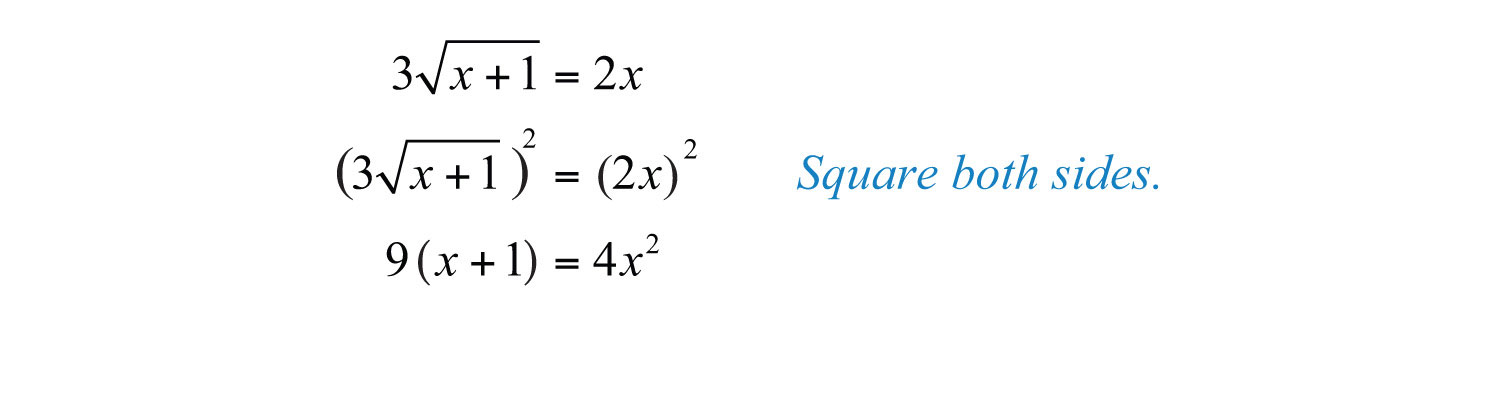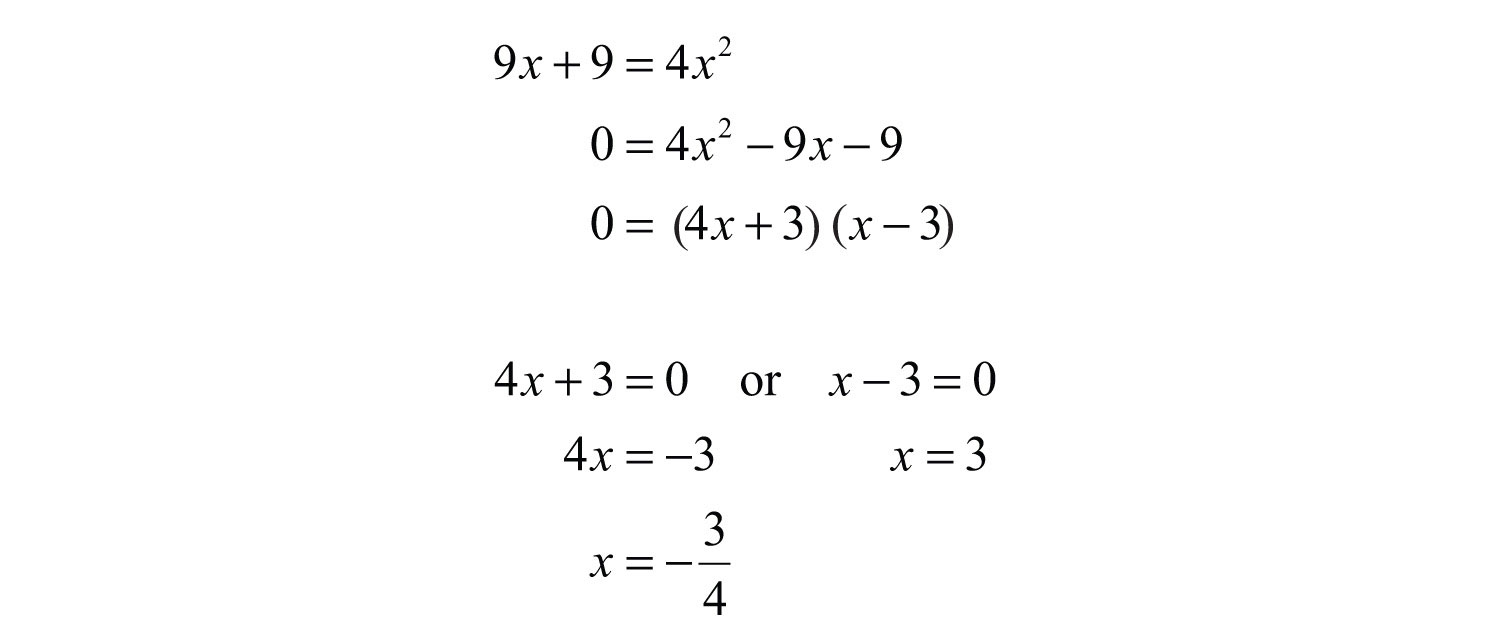Since we squared both sides, we must check our solutions.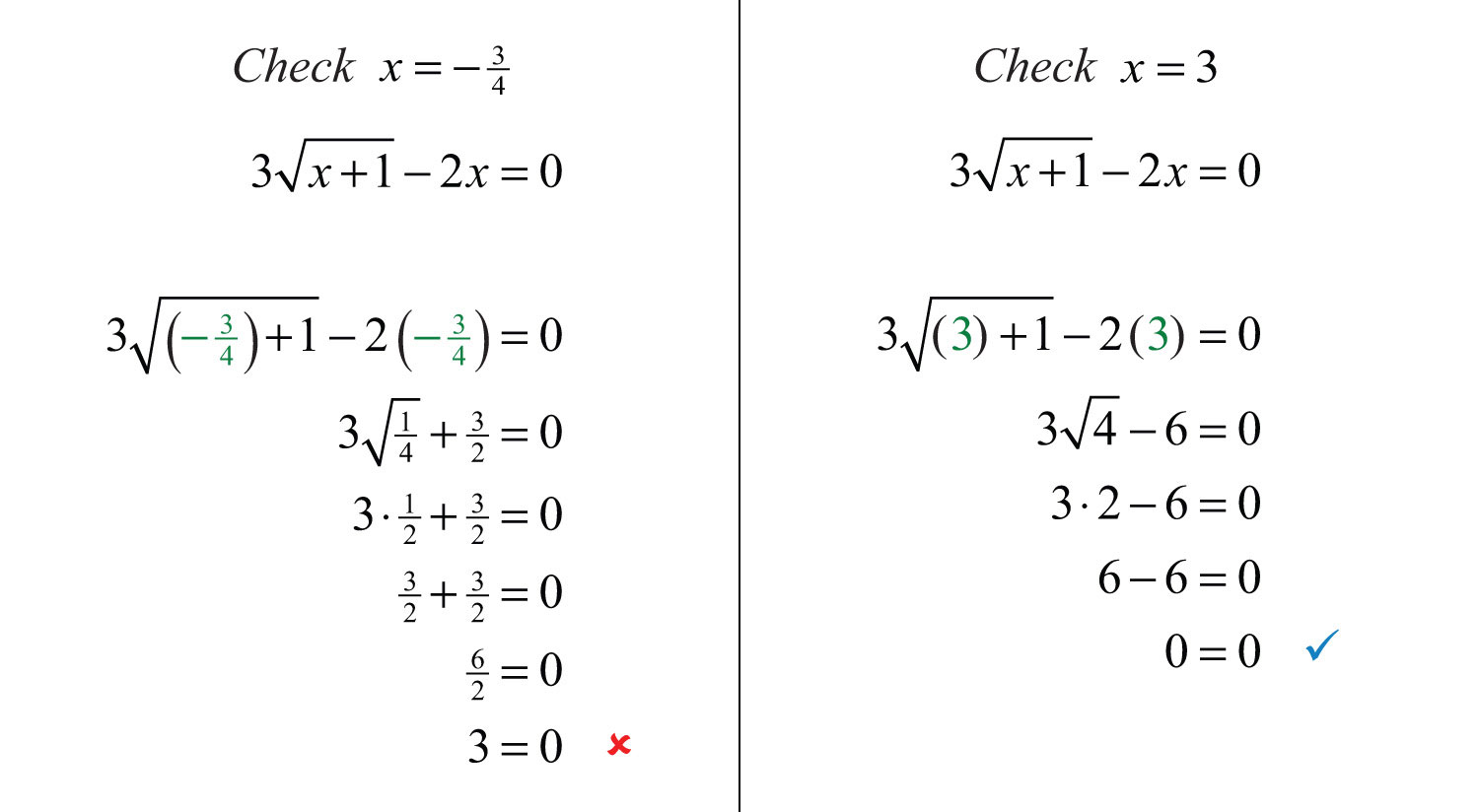After checking, we can see that $x=−34$ was extraneous.

Sometimes both of the possible solutions are extraneous.

Example 5: Solve: $4−11x−x+2=0$.

Solution: Begin by isolating the radical.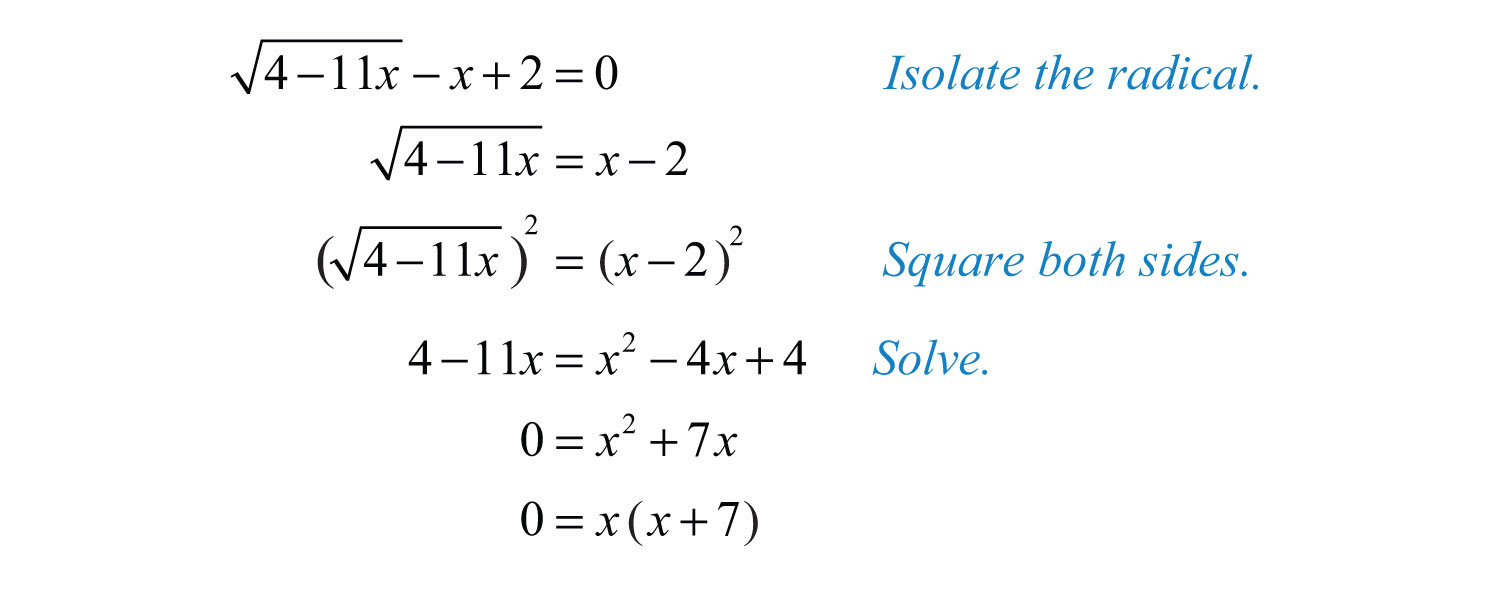Since we squared both sides, we must check our solutions.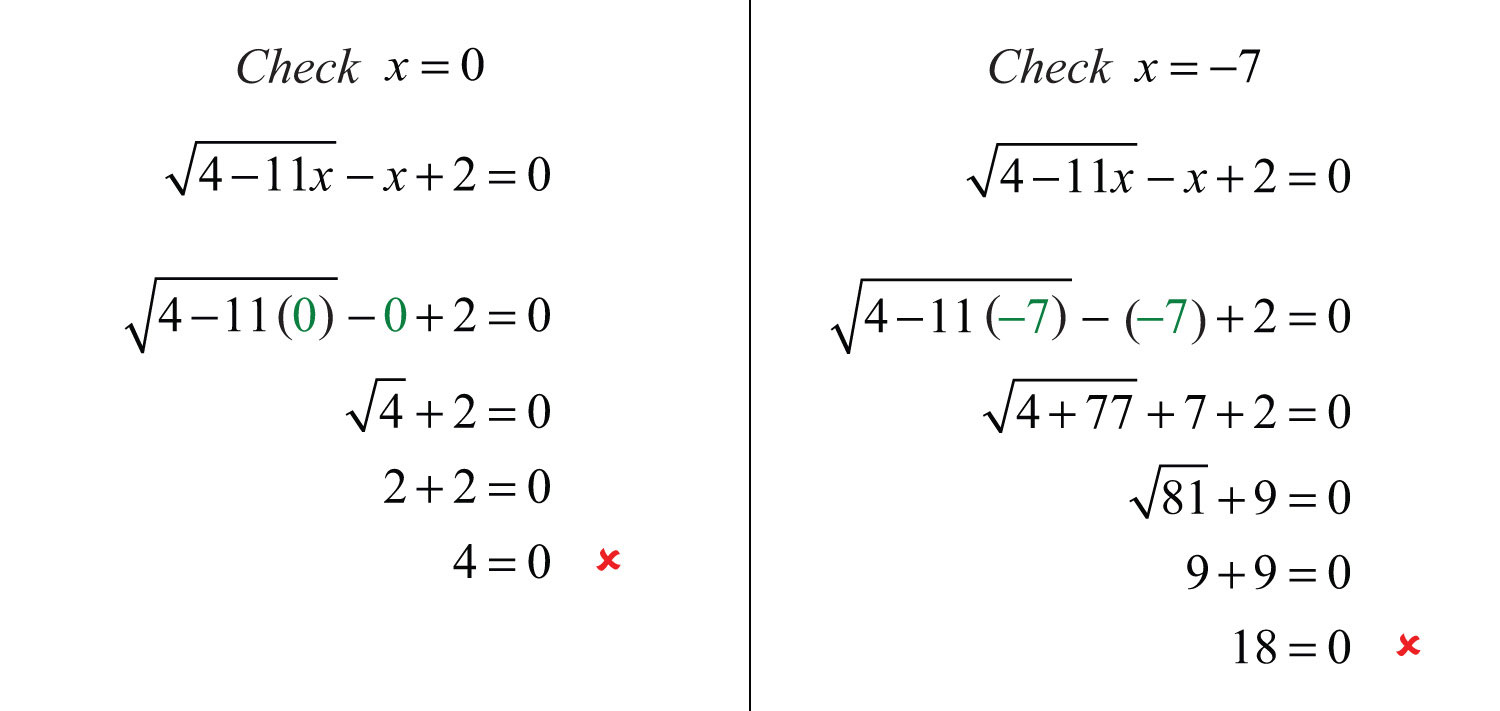Since both possible solutions are extraneous, the equation has no solution.

The squaring property of equality extends to any positive integer power n. Given real numbers a and b, we have the following:This is often referred to as the power property of equalityGiven any positive integer n and real numbers a and b, where $a=b$, then $an=bn$.. Use this property, along with the fact that $(an)n=ann=a$, when a is positive, to solve radical equations with indices greater than 2.

Example 6: Solve: $x2+43−2=0$.

Solution: Isolate the radical and then cube both sides of the equation.Check.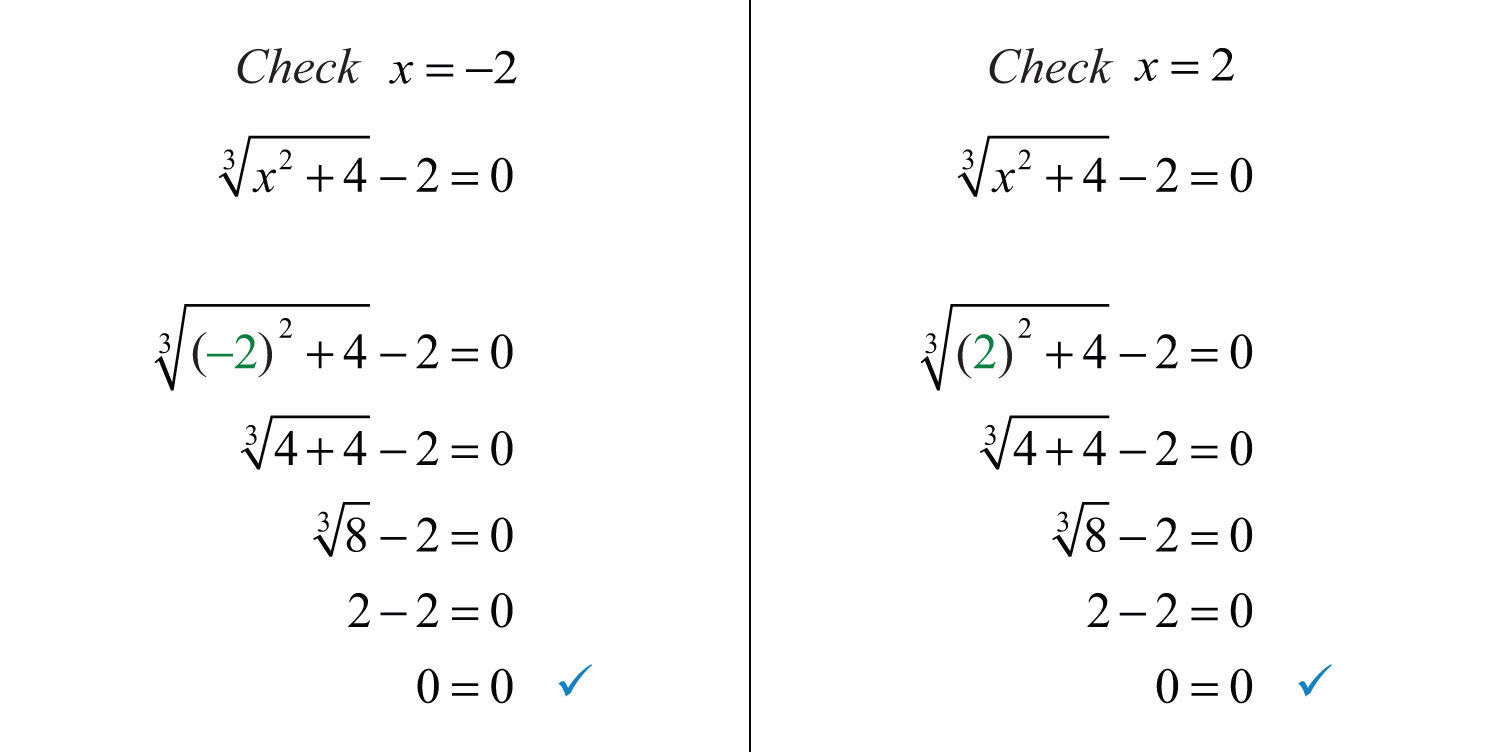Answer: The solutions are −2 and 2.

Try this! Solve: $2x−1+2=x$.

Answer: $x=5$ ($x=1$ is extraneous)

### Video Solution

(click to see video)

It may be the case that the equation has two radical expressions.

Example 7: Solve: $3x−4=2x+9$.

Solution: Both radicals are considered isolated on separate sides of the equation.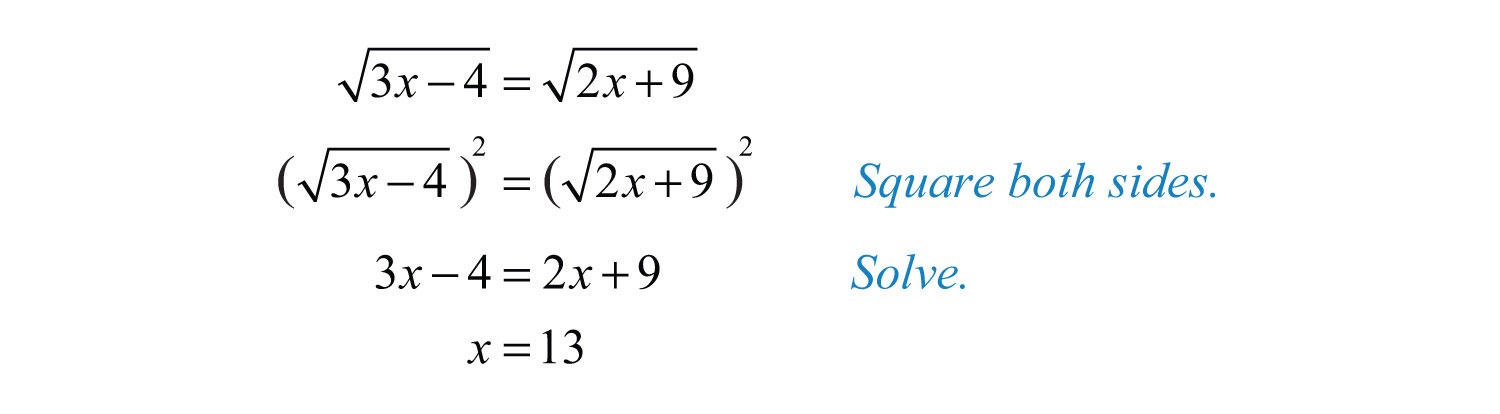Check $x=13$.Example 8: Solve: $x2+x−143=x+503$.

Solution: Eliminate the radicals by cubing both sides.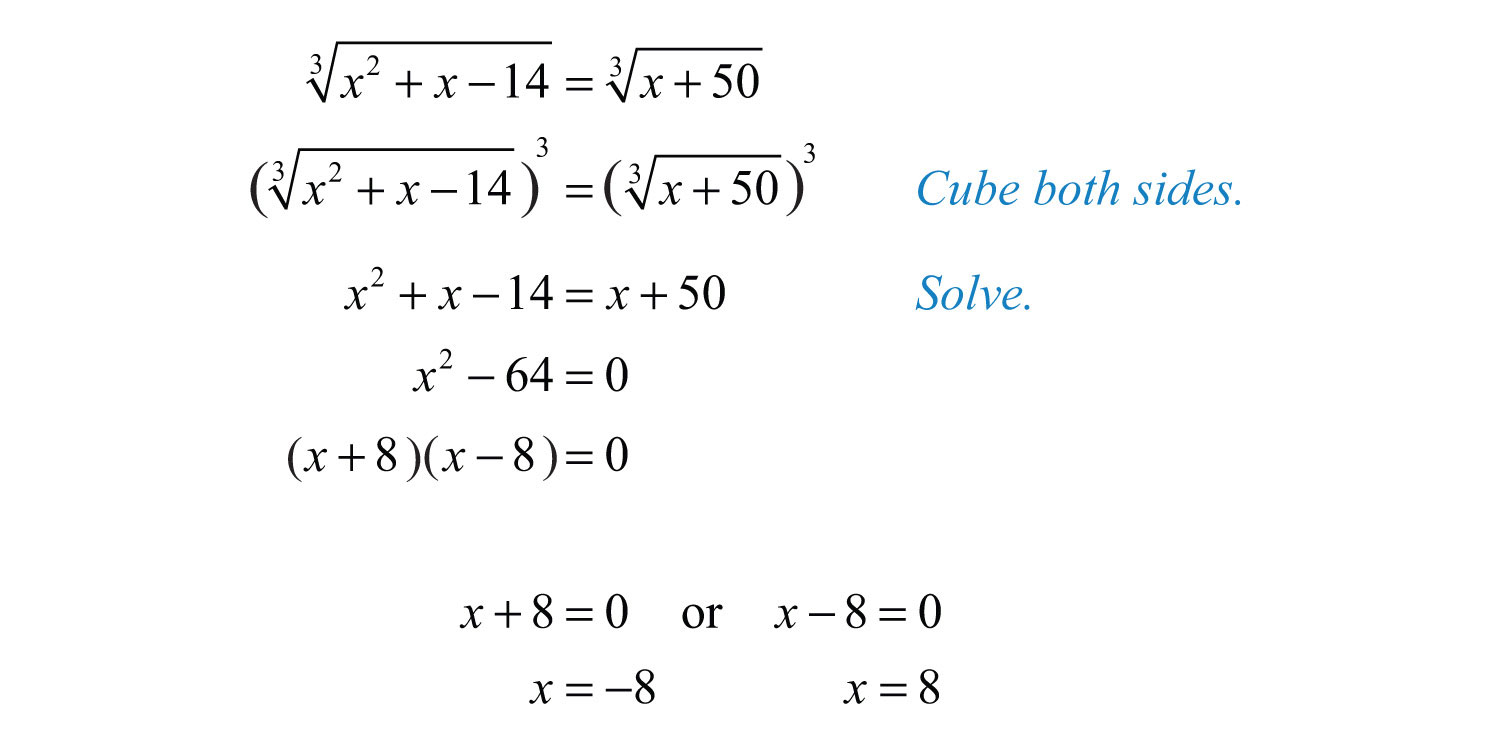Check.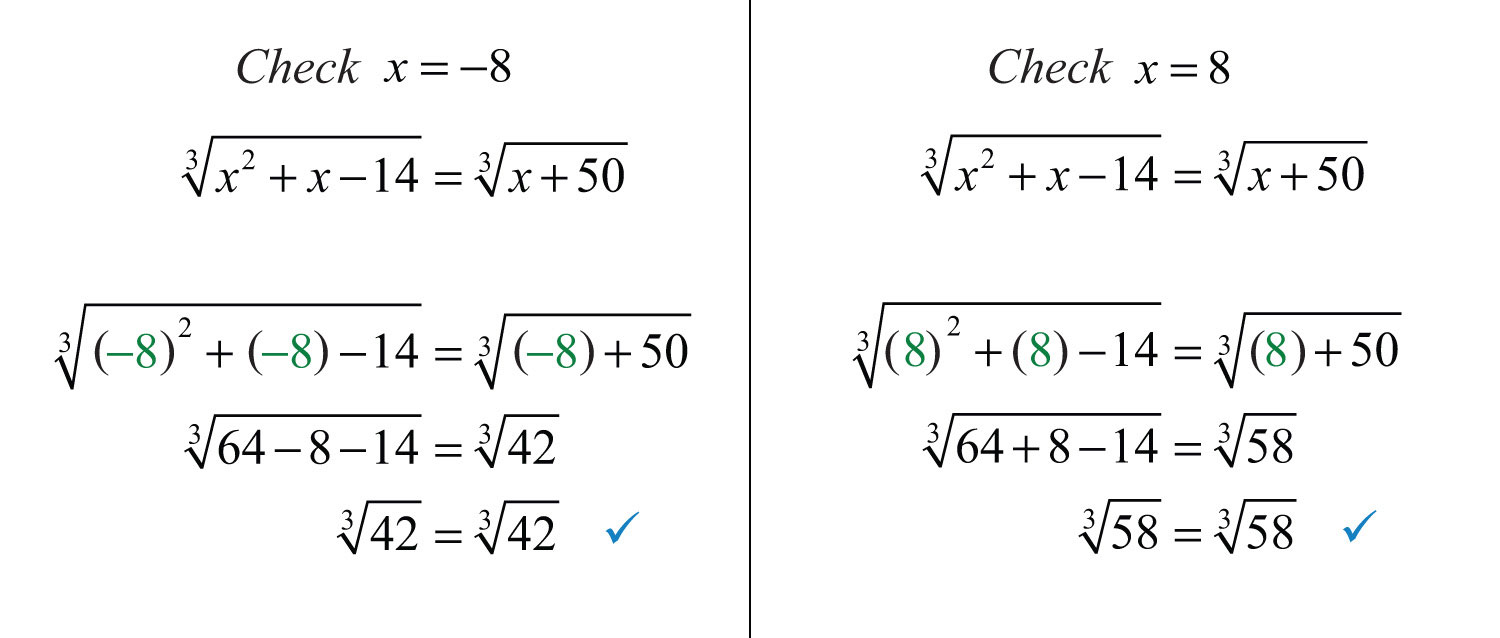Answer: The solutions are −8 and 8.

We will learn how to solve some of the more advanced radical equations in the next course, Intermediate Algebra.

Try this! Solve: $3x+1=2x−3$.

### Video Solution

(click to see video)

### Key Takeaways

• Solve equations involving square roots by first isolating the radical and then squaring both sides. Squaring a square root eliminates the radical, leaving us with an equation that can be solved using the techniques learned earlier in our study of algebra. However, squaring both sides of an equation introduces the possibility of extraneous solutions, so check your answers in the original equation.
• Solve equations involving cube roots by first isolating the radical and then cubing both sides. This eliminates the radical and results in an equation that may be solved with techniques you have already mastered.

### Topic Exercises

Solve.

1. $x=2$

2. $x=7$

3. $x+7=8$

4. $x+4=9$

5. $x+6=3$

6. $x+2=1$

7. $5x−1=0$

8. $3x−2=0$

9. $x−3=3$

10. $x+5=6$

11. $3x+1=2$

12. $5x−4=4$

13. $7x+4+6=11$

14. $3x−5+9=14$

15. $2x−1−3=0$

16. $3x+1−2=0$

17. $x3=2$

18. $x3=5$

19. $2x+93=3$

20. $4x−113=1$

21. $5x+73+3=1$

22. $3x−63+5=2$

23. $2 x+23−1=0$

24. $2 2x−33−1=0$

25. $8x+11=3x+1$

26. $23x−4=2(3x+1)$

27. $2(x+10)=7x−15$

28. $5(x−4)=x+4$

29. $5x−23=4x3$

30. $9(x−1)3=3(x+7)3$

31. $3x+13=2(x−1)3$

32. $9x3=3(x−6)3$

33. $4x+21=x$

34. $8x+9=x$

35. $4(2x−3)=x$

36. $3(4x−9)=x$

37. $2x−1=x$

38. $32x−9=x$

39. $9x+9=x+1$

40. $3x+10=x+4$

41. $x−1=x−3$

42. $2x−5=x−4$

43. $16−3x=x−6$

44. $7−3x=x−3$

45. $32x+10=x+9$

46. $22x+5=x+4$

47. $3x−1−1=x$

48. $22x+2−1=x$

49. $10x+41−5=x$

50. $6(x+3)−3=x$

51. $8x2−4x+1=2x$

52. $18x2−6x+1=3x$

53. $5x+2=x+8$

54. $42(x+1)=x+7$

55. $x2−25=x$

56. $x2+9=x$

57. $3+6x−11=x$

58. $2+9x−8=x$

59. $4x+25−x=7$

60. $8x+73−x=10$

61. $24x+3−3=2x$

62. $26x+3−3=3x$

63. $2x−4=14−10x$

64. $3x−6=33−24x$

65. $x2−243=1$

66. $x2−543=3$

67. $x2+6x3+1=4$

68. $x2+2x3+5=7$

69. $25x2−10x−73=−2$

70. $9x2−12x−233=−3$

71. $2x2−15x+25=(x+5)(x−5)$

72. $x2−4x+4=x(5−x)$

73. $2(x2+3x−20)3=(x+3)23$

74. $3x2+3x+403=(x−5)23$

75. $x1/2−10=0$

76. $x1/2−6=0$

77. $x1/3+2=0$

78. $x1/3+4=0$

79. $(x−1)1/2−3=0$

80. $(x+2)1/2−6=0$

81. $(2x−1)1/3+3=0$

82. $(3x−1)1/3−2=0$

83. $(4x+15)1/2−2x=0$

84. $(3x+2)1/2−3x=0$

85. $(2x+12)1/2−x=6$

86. $(4x+36)1/2−x=9$

87. $2(5x+26)1/2=x+10$

88. $3(x−1)1/2=x+1$

89. The square root of 1 less than twice a number is equal to 2 less than the number. Find the number.

90. The square root of 4 less than twice a number is equal to 6 less than the number. Find the number.

91. The square root of twice a number is equal to one-half of that number. Find the number.

92. The square root of twice a number is equal to one-third of that number. Find the number.

93. The distance, d, measured in miles, a person can see an object is given by the formula $d=6h2$

where h represents the person’s height above sea level, measured in feet. How high must a person be to see an object 5 miles away?

94. The current, I, measured in amperes, is given by the formula $I=PR$

where P is the power usage, measured in watts, and R is the resistance, measured in ohms. If a light bulb requires 1/2 ampere of current and uses 60 watts of power, then what is the resistance of the bulb?

The period, T, of a pendulum in seconds is given by the formula $T=2πL32$

where L represents the length in feet. For each problem below, calculate the length of a pendulum, given the period. Give the exact value and the approximate value rounded off to the nearest tenth of a foot.

95. 1 second

96. 2 seconds

97. 1/2 second

98. 1/3 second

The time, t, in seconds an object is in free fall is given by the formula $t=s4$

where s represents the distance in feet the object has fallen. For each problem below, calculate the distance an object falls, given the amount of time.

99. 1 second

100. 2 seconds

101. 1/2 second

102. 1/4 second

The x-intercepts for any graph have the form (x, 0), where x is a real number. Therefore, to find x-intercepts, set y = 0 and solve for x. Find the x-intercepts for each of the following.

103. $y=x−3−1$

104. $y=x+2−3$

105. $y=x−13+2$

106. $y=x+13−3$

Part B: Discussion Board

107. Discuss reasons why we sometimes obtain extraneous solutions when solving radical equations. Are there ever any conditions where we do not need to check for extraneous solutions? Why?

1: 4

3: 1

5: Ø

7: 1/25

9: 12

11: 1

13: 3

15: 13/4

17: 8

19: 9

21: −3

23: −15/8

25: 2

27: 7

29: 2

31: −3

33: 7

35: 2, 6

37: 2

39: −1, 8

41: 5

43: Ø

45: −3, 3

47: 2, 5

49: −4, −4

51: 1/2

53: 2, 7

55: Ø

57: 10

59: −6, −4

61: −1/2, 3/2

63: Ø

65: −5, 5

67: −9, 3

69: 1/5

71: 5, 10

73: −7, 7

75: 100

77: −8

79: 10

81: −13

83: 5/2

85: −6, −4

87: −2, 2

89: 5

91: 8

93: $1623$ feet

95: $8/π2≈0.8$ foot

97: $2/π2≈0.2$ foot

99: 16 feet

101: 4 feet

103: (4, 0)

105: (−7, 0)